# FPGA-Based Minimum-Variance Distortionless-Response (MVDR) Beamformer

This example shows how to implement a minimum-variance distortionless-response (MVDR) beamformer suitable for hardware.

### MVDR Objective

The MVDR beamformer preserves the gain in the direction of arrival of a desired signal and attenuates interference from other directions , .

Given readings from a sensor array, such as the uniform linear array (ULA) in the following diagram, form data matrix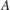from samples of the array, where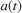is an-by-1 column vector of readings from the array sampled at time, and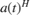is one row of matrix. Many more samples are taken than there are elements in the array. This results in the number of rows inbeing much greater than the number of columns. An estimate of the covariance matrix is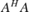, where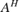is the Hermitian or complex-conjugate transpose of.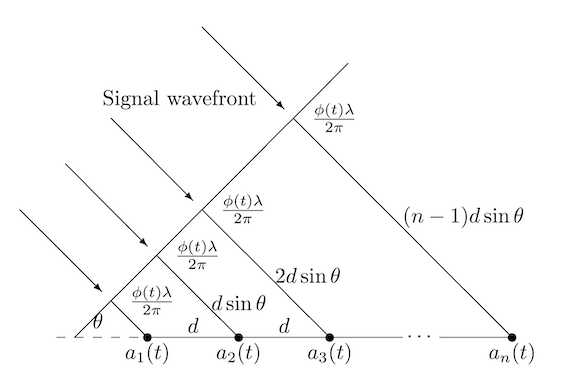Compute the MVDR beamformer response by solving the following equation for, where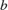is a steering vector pointing in the direction of the desired signal.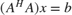The MVDR weight vectoris computed fromandusing the following equation, which normalizesto preserve the gain in the direction of arrival of the desired signal.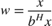The MVDR system response is the inner product between the MVDR weight vectorand a current sample from the sensor array.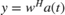### HDL-Optimized MVDR

The three equations in the previous section are implemented by the three primary blocks in the following model. The rate changes give the matrix solve additional clock cycles to update before the next input sample. The number of clock cycles between a valid input and when the complex matrix solve block is ready is twice its input wordlength to allow time for CORDIC iterations, plus 15 cycles for internal delays.

load_system('MVDRBeamformerHDLOptimizedModel'); open_system('MVDRBeamformerHDLOptimizedModel/MVDR - HDL Optimized')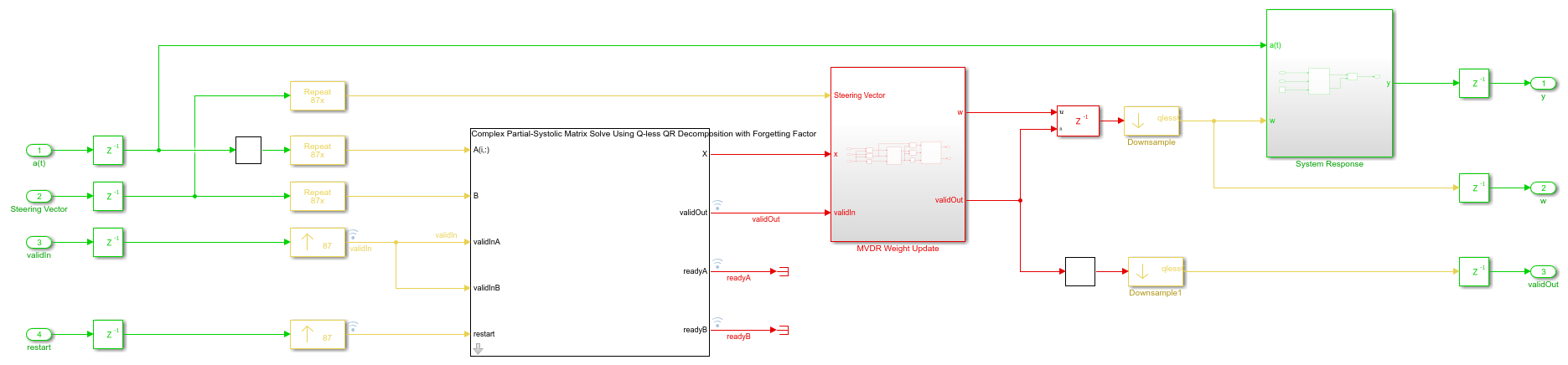Instead of forming data matrixand computing the Cholesky factorization of covariance matrix, the upper-triangular matrix of the QR decomposition ofis computed directly and updated as each data vectorstreams in from the sensor array. Because the data is updated indefinitely, a forgetting factor is applied after each factorization. To integrate with an equivalent of a matrix ofrows, the forgetting factorshould be set to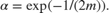This example simulates the equivalent of a matrix with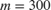rows, so the forgetting factor is set to 0.9983.

The Complex Partial-Systolic Matrix Solve Using Q-less QR Decomposition with Forgetting Factor block is implemented using the method found in . The upper-triangular matrix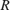from the QR decomposition ofis identical to the Cholesky factorization ofexcept the signs of values on the diagonal. Solving the matrix equationby computing the Cholesky factorization ofis not as efficient or as numerically sound as computing the QR decomposition ofdirectly .

### Run Model

Open and simulate the model.

open_system('MVDRBeamformerHDLOptimizedModel') scopes = find_system('MVDRBeamformerHDLOptimizedModel','BlockType','Scope'); close_system(scopes);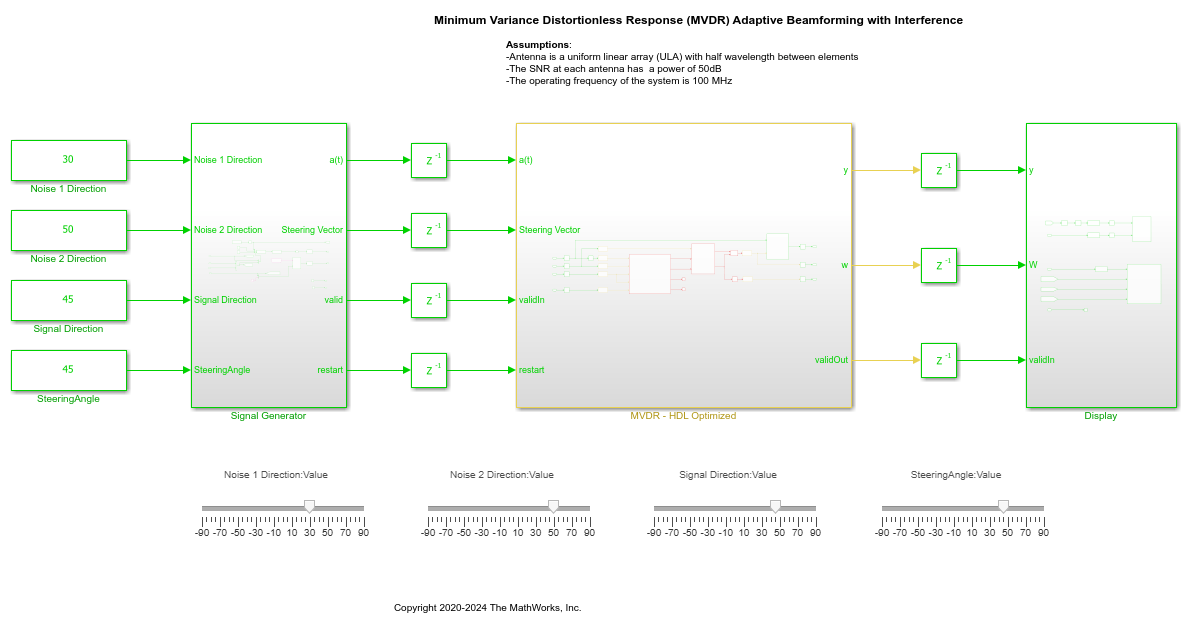As the model is simulating, you can adjust the signal direction, steering angle and noise directions by dragging the sliders, or by editing the constant values.

When the signal direction and steering angle are aligned as indicated by the blue and green lines, you can see that the beam pattern has a gain of 0 dB. The noise sources are nulled as indicated by the red lines.The desired pulse appears when the noise sources are nulled. This example simulates with the same latency as the hardware, so you can see the signal settle over time as the simulation starts and when the directions are changed.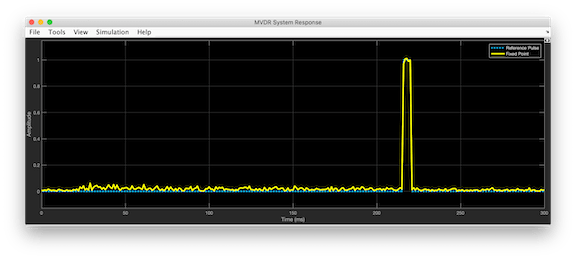### Set Parameters

The parameters for the beamformer are set in the model workspace. You can modify the parameters by editing and running the setMVDRExampleModelWorkspace function.

### References

 V. Behar et al. "Parameter Optimization of the adaptive MVDR QR-based beamformer for jamming and multipath supression in GPS/GLONASS receivers". In: Proc. 16th Saint Petersburg International Conference on Integrated Navigation systems. Saint Petersburg, Russia, May 2009, pp. 325--334.

 Jack Capon. "High-resolution frequency-wavenumber spectrum analysis". In: vol. 57. 1969, pp. 1408--1418.

 C.M. Rader. "VLSI Systolic Arrays for Adaptive Nulling". In: IEEE Signal Processing Magazine (July 1996), pp. 29--49.

 Charles F. Van Loan. Introduction to Scientific Computing: A Matrix-Vector Approach Using Matlab. Second edition. Prentice-Hall, 2000. isbn: 0-13-949157-0.